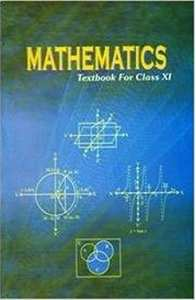Advertisement Remove all ads

# NCERT solutions for Class 11 Mathematics Textbook chapter 8 - Binomial Theorem [Latest edition]

#### ChaptersAdvertisement Remove all ads
Advertisement Remove all ads
Advertisement Remove all ads

## Chapter 8: Binomial Theorem

Advertisement Remove all ads
[Pages 166 - 167]

### NCERT solutions for Class 11 Mathematics Textbook Chapter 8 Binomial Theorem[Pages 166 - 167]

Q 1 | Page 166

Expand the expression (1– 2x)5

Q 1 | Page 166

Expand the expression (1– 2x)5

Q 2 | Page 166

Expand the expression (2/x - x/2)^5

Q 3 | Page 166

Expand the expression (2x – 3)6

Q 4 | Page 167

Expand the expression (x/3 + 1/x)^5

Q 5 | Page 167

Expand (x + 1/x)^6

Q 6 | Page 167

Using Binomial Theorem, evaluate (96)3

Q 7 | Page 167

Using Binomial Theorem, evaluate (102)5

Q 8 | Page 167

Using Binomial Theorem, evaluate (101)4

Q 9 | Page 167

Using Binomial Theorem, evaluate (99)5

Q 10 | Page 167

Using Binomial Theorem, indicate which number is larger (1.1)10000 or 1000.

Q 11 | Page 167

Find (a + b)4 – (a – b)4. Hence, evaluate (sqrt3 + sqrt2)^4 - (sqrt3 - sqrt2)^4

Q 12 | Page 167

Find (x + 1)6 + (x – 1)6. Hence or otherwise evaluate (sqrt2 + 1)^6 + (sqrt2 -1)^6

Q 13 | Page 167

Show that 9n+1 – 8n – 9 is divisible by 64, whenever n is a positive integer.

Q 14 | Page 167

Prove that sum_(r-0)^n 3^r  ""^nC_r = 4^n

[Page 171]

### NCERT solutions for Class 11 Mathematics Textbook Chapter 8 Binomial Theorem[Page 171]

Q 1 | Page 171

Find the coefficient of x5 in (x + 3)8

Q 2 | Page 171

Find the coefficient of a5b7 in (a – 2b)12

Q 3 | Page 171

Write the general term in the expansion of (x2 – y)6

Q 4 | Page 171

Write the general term in the expansion of (x2 – yx)12x ≠ 0

Q 5 | Page 171

Find the 4th term in the expansion of (x – 2y)12 .

Q 6 | Page 171

Find the 13th term in the expansion of (9x - 1/(3sqrtx))^18 , x != 0

Q 7 | Page 171

Find the middle terms in the expansions of  (3 - x^3/6)^7

Q 8 | Page 171

Find the middle terms in the expansions of (x/3 + 9y)^10

Q 9 | Page 171

In the expansion of (1 + a)m + n, prove that coefficients of am and an are equal.

Q 10 | Page 171

The coefficients of the (r – 1)thrth and (r + 1)th terms in the expansion of (x + 1)n are in the ratio 1:3:5. Find n and r.

Q 11 | Page 171

Prove that the coefficient of xn in the expansion of (1 + x)2n is twice the coefficient of xn in the expansion of (1 + x)2n–1 .

Q 12 | Page 171

Find a positive value of m for which the coefficient of x2 in the expansion

(1 + x)m is 6

[Pages 175 - 176]

### NCERT solutions for Class 11 Mathematics Textbook Chapter 8 Binomial Theorem[Pages 175 - 176]

Q 1 | Page 175

Find ab and n in the expansion of (a + b)n if the first three terms of the expansion are 729, 7290 and 30375, respectively.

Q 1 | Page 175

Find a if the coefficients of x2 and x3 in the expansion of (3 + ax)9 are equal.

Q 3 | Page 175

Find the coefficient of x5 in the product (1 + 2x)6 (1 – x)7 using binomial theorem.

Q 4 | Page 175

If a and b are distinct integers, prove that a – b is a factor of an – bn, whenever n is a positive integer.

[Hint: write an = (a – b b)n and expand]

Q 5 | Page 175

Evaluate (sqrt3  +sqrt2)^6 - (sqrt3 - sqrt2)^6

Q 6 | Page 175

Find the value of (a^2 + sqrt(a^2 - 1))^4 + (a^2 - sqrt(a^2 -1))^4

Q 7 | Page 175

Find an approximation of (0.99)5 using the first three terms of its expansion.

Q 8 | Page 175

Find n, if the ratio of the fifth term from the beginning to the fifth term from the end in the expansion of (root4 2 + 1/ root4 3)^n " is " sqrt6 : 1

Q 9 | Page 176

Expand using Binomial Theorem (1+ x/2 - 2/x)^4, x != 0

Q 10 | Page 176

Find the expansion of (3x2 – 2ax + 3a2)3 using binomial theorem.

## Chapter 8: Binomial Theorem## NCERT solutions for Class 11 Mathematics Textbook chapter 8 - Binomial Theorem

NCERT solutions for Class 11 Mathematics Textbook chapter 8 (Binomial Theorem) include all questions with solution and detail explanation. This will clear students doubts about any question and improve application skills while preparing for board exams. The detailed, step-by-step solutions will help you understand the concepts better and clear your confusions, if any. Shaalaa.com has the CBSE Class 11 Mathematics Textbook solutions in a manner that help students grasp basic concepts better and faster.

Further, we at Shaalaa.com provide such solutions so that students can prepare for written exams. NCERT textbook solutions can be a core help for self-study and acts as a perfect self-help guidance for students.

Concepts covered in Class 11 Mathematics Textbook chapter 8 Binomial Theorem are Binomial Theorem for Positive Integral Indices, General and Middle Terms, Introduction of Binomial Theorem, Proof of Binomial Therom by Pattern, Proof of Binomial Therom by Combination, Rth Term from End, Simple Applications of Binomial Theorem.

Using NCERT Class 11 solutions Binomial Theorem exercise by students are an easy way to prepare for the exams, as they involve solutions arranged chapter-wise also page wise. The questions involved in NCERT Solutions are important questions that can be asked in the final exam. Maximum students of CBSE Class 11 prefer NCERT Textbook Solutions to score more in exam.

Get the free view of chapter 8 Binomial Theorem Class 11 extra questions for Class 11 Mathematics Textbook and can use Shaalaa.com to keep it handy for your exam preparation

Advertisement Remove all ads
Share
Notifications

View all notifications

Forgot password?